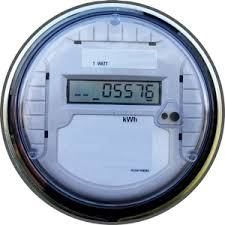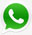News

Home > News

# How to classify electric energy meters?(2)

Apr 15, 20174. According to connection modes, electric energy meters can be classified as connection through transformers and direct connection. Current from connection through transformers: 3X0.3 (1.2) A, 3X0.5 (2) A, 3X1.5 (6) A, and 3X5 (10) A, etc.

Current from direct connection: 3X5 (20) A, 3X10 (40) A, and 3X20 (80) A, etc. Now current specifications of energy meters are mostly wide load. Take 3X5 (20) A as an example. 5 represents rated current and (20) stands for maximum current rating, four times the rated current.

5. According to measuring accuracy, electric energy meters can be classified as: watt-hour: class 0.2S, 0.5S, 0.5, 1.0 and 2.0; var-hour: class 0.5, 1.0 and 2.0.

6. According to purposes, electric energy meters can be divided into single phase energy meter, three-phase watt-hour energy meter, three-phase var-hour energy meter, maximum demand meter, multi-rate energy meter (time-based), multifunctional watthour meter, loss-of-voltage timer, copper loss meter, iron loss meter and prepayment energy meter, among others.

••